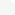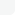## Categories# Video Training →The Stata Omnibus Regression and Modelling with Stata

MP4 | Video: h264, 1920x1080 | Audio: AAC, 44.1 KHz, 2 Ch
November 2020 | ISBN: 9781800563261 | English
Duration: 137 Lessons (14h 13m) | Size: 2.8 GB

Get access to a fully updated course that simplifies the concept of linear and non-linear regression, regression modeling, and Stata
Learn
Distinguish between linear and non-linear regression analysis
Model non-linear relationships in linear regression
Perform regression modeling and data visualization in Stata
Interpret and analyze regression output from ordinary least squares (OLS)
Understand the basic statistical intuition behind non-linear regression
Work with dummy variables in logit and probit regression
Learning and applying new statistical techniques can be a daunting experience, especially when working with complex datasets that do not allow for easy click-and-go analysis but require a deeper level of understanding of analytical methodologies.
This course will introduce you to data analytics using Stata and prepare you to handle real-life complex datasets with ease. Starting with an overview of the learning objectives, it takes you straight into the concepts of linear regression, non-linear regression, and regression modeling. Once you've got a comprehensive introduction to Stata and its various uses in modern data analysis, you'll discover how you can use Stata extensively for manipulating, exploring, visualizing, and modeling complex types of data. As you advance, you'll also learn how to use the Analysis of Variance (ANOVA) test to find out significant differences between different groups. You'll then explore the functionality and application of ordinary least squares (OLS) and study how logit and probit models work. Finally, you'll cover some simulation techniques and features of the count data model and get to grips with survival and panel data analysis.
By the end of this course, you'll have developed the skills and ability to engage with Stata for handling complex data analytics.
All the code files and resources for this course are available at
``https://github.com/PacktPublishing/The-STATA-OMNIBUS-Regression-and-Modelling-with-STATA``

Features
View, explore, analyze, manipulate, and visualize data with Stata
Understand linear regression and non-linear regression analysis
Discover tips and techniques for building regression models

## Related News## Information

Users of Guests are not allowed to comment this publication.